# Mixture distributions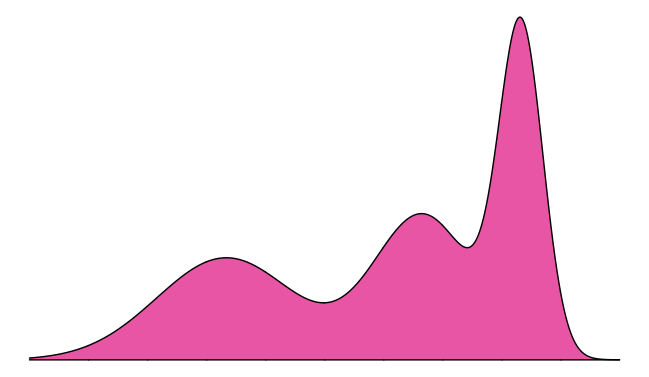UPDATE: For a clean implementation of mixture distributions, check out the distplyr R package.

This tutorial introduces the concept of a mixture distribution. We’ll look at a basic example first, using intuition, and then describe mixture distributions mathematically. See the very end for a summary of the learning points.

## Intuition

Let’s start by looking at a basic experiment:

1. Flip a coin.
2. If the outcome is heads, generate a N(0,1) random variable. If the outcome is tails, generate a N(4,1) random variable. We’ll let $$X$$ denote the final result.

$$X$$ is a random variable with some distribution (spoiler: it’s a mixture distribution). Let’s perform the experiment 1000 times to get 1000 realizations of $$X$$, and make a histogram to get a sense of the distribution $$X$$ follows. To make sure the histogram represents an estimate of the density, we’ll make sure the area of the bars add to 1 (with the ..density.. option).

library(tidyverse)
set.seed(44)
X <- numeric(0)
coin <- integer(0)
for (i in 1:1000) {
coin[i] <- rbinom(1, size = 1, prob = 0.5)  # flip a coin. 0=heads, 1=tails.
if (coin[i] == 0) {   # heads
X[i] <- rnorm(1, mean = 0, sd = 1)
} else {           # tails
X[i] <- rnorm(1, mean = 4, sd = 1)
}
}
(p <- qplot(X, ..density.., geom = "histogram", bins = 30))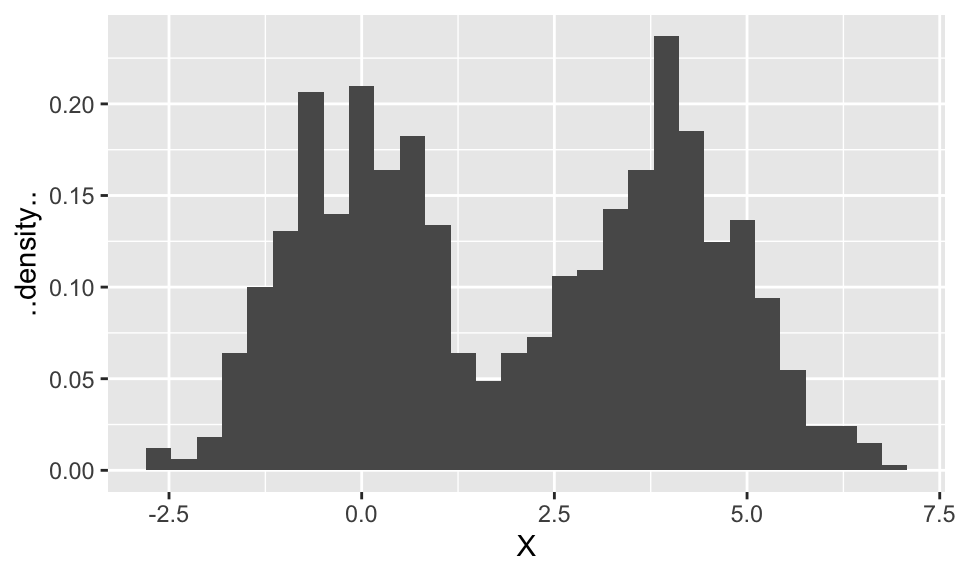Let’s try to reason our way to figuring out the overall density. Keep in mind that this density (like all densities) is one curve. We’ll say we’ve succeeded at finding the density if our density is close to the histogram.

It looks like the histogram is made up of two normal distributions “superimposed”. These ought to be related to the N(0,1) and N(4,1) distributions, so to start, let’s plot these two Gaussian densities overtop of the histogram.

tibble(X = X) %>%
ggplot(aes(X)) +
geom_histogram(aes(y = ..density..), bins = 30) +
stat_function(fun = function(x) dnorm(x, mean = 0, sd = 1),
mapping = aes(colour = "Heads")) +
stat_function(fun = function(x) dnorm(x, mean = 4, sd = 1),
mapping = aes(colour = "Tails")) +
scale_color_discrete("Coin Flip")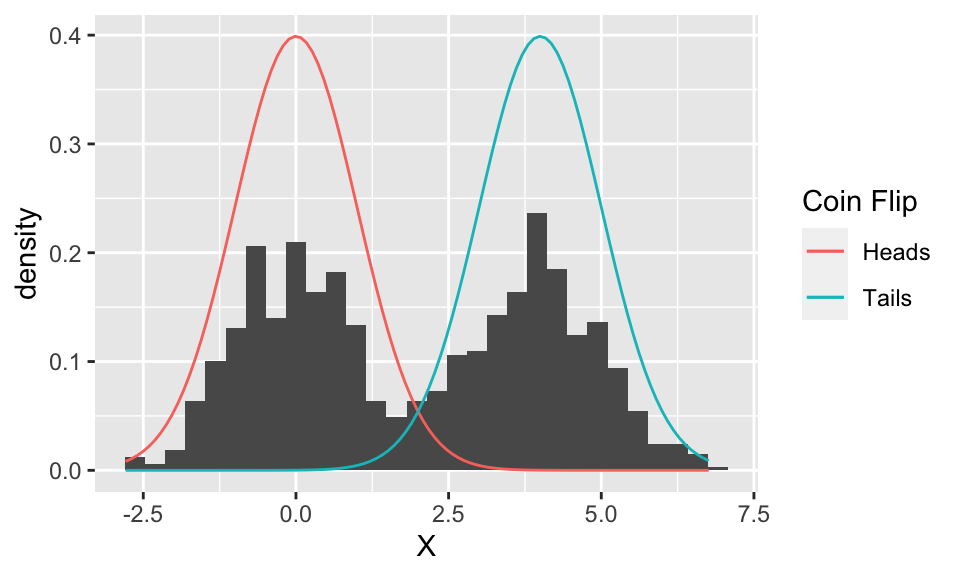Well, the two Gaussian distributions are in the correct location, and it even looks like they have the correct spread, but they’re too tall.

Something to note at this point: the two curves plotted above are separate (component) distributions. We’re trying to figure out the distribution of $$X$$ – which, again, is a single curve, and is estimated by the histogram. At this point, we only suspect that the distribution of $$X$$ is some combination of these two Gaussian distributions.

So, why are the Gaussian curves too tall? Because each one represents the distribution if we only ever flip either heads or tails (for example, the red distribution happens when we only ever flip heads). But since we flip heads half of the time, and tails half of the time, these probabilities (more accurately, densities) ought to be reduced by half. Let’s add these “semi” component distributions to the plot:

(p <- tibble(X = X) %>%
ggplot(aes(X)) +
geom_histogram(aes(y = ..density..), bins = 30) +
stat_function(fun = function(x) dnorm(x, mean = 0, sd = 1)*0.5,
mapping = aes(colour = "Heads", linetype = "Semi")) +
stat_function(fun = function(x) dnorm(x, mean = 4, sd = 1)*0.5,
mapping = aes(colour = "Tails", linetype = "Semi")) +
stat_function(fun = function(x) dnorm(x, mean = 0, sd = 1),
mapping = aes(colour = "Heads", linetype = "Full")) +
stat_function(fun = function(x) dnorm(x, mean = 4, sd = 1),
mapping = aes(colour = "Tails", linetype = "Full")) +
scale_color_discrete("Coin Flip") +
scale_linetype_discrete("Distribution"))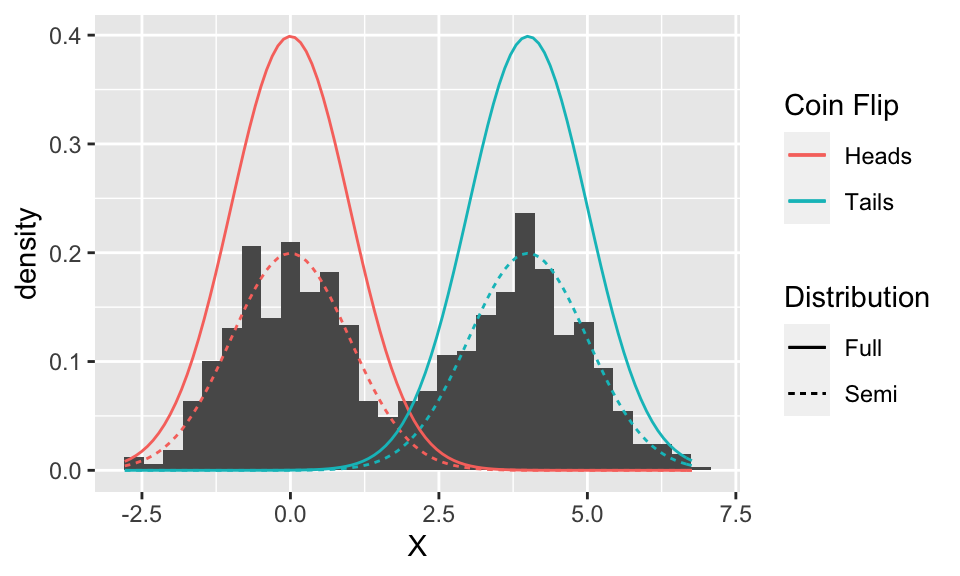Looks like they line up quite nicely!

But these two curves are still separate – we need one overall curve if we are to find the distribution of $$X$$. So we need to combine them somehow. It might look at first that we can just take the upper-most of the ‘semi’ curves (i.e., the maximum of the two), but looking in between the two curves reveals that the histogram is actually larger than either curve here. It turns out that the two ‘semi’ curves are added to get the final curve:

p + stat_function(fun = function(x) dnorm(x, mean = 0, sd = 1) * 0.5 +
dnorm(x, mean = 4, sd = 1) * 0.5,
mapping = aes(linetype = "Full"))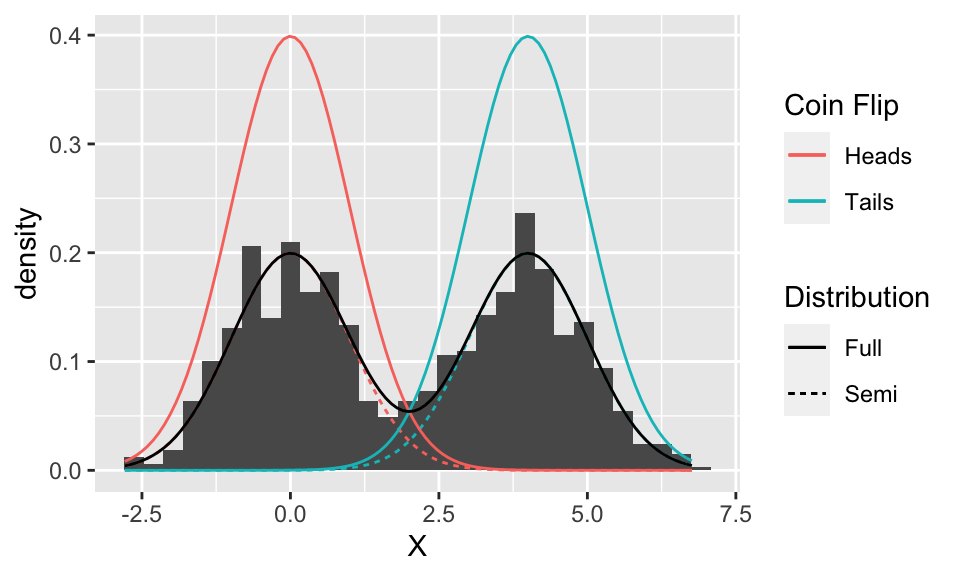The intuition behind adding the densities is that an outcome for $$X$$ comes from both components, so both contribute some density.

Even though the random variable $$X$$ is made up of two components, at the end of the day, it’s still overall just a random variable with some density. And like all densities, the density of $$X$$ is just one curve. But, this density happens to be made up of the components, as we’ll see next.

## General Scenario

The two normal distributions from above are called component distributions. In general, we can have any number of these (not just two) to make a mixture distribution. And, instead of selecting the component distribution with coin tosses, they’re chosen according to some generic probabilities called the mixture probabilities.

In general, here’s how we make a mixture distribution with $$K$$ component Gaussian distributions with densities $$\phi_1(x), \ldots, \phi_K(x)$$:

1. Choose one of the $$K$$ components, randomly, with mixture probabilities $$\pi_1, \ldots, \pi_K$$ (which, by necessity, add to 1).
2. Generate a random variable from the selected component distribution. Call the result $$X$$.

Note: we can use more than just Gaussian component distributions! But this tutorial won’t demonstrate that.

That’s how we generate a random variable with a mixture distribution, but what’s its density? We can derive that by the law of total probability. Let $$C$$ be the selected component number; then the component distributions are actually the distribution of $$X$$ conditional on the component number. We get: $f_X\left(x\right) = \sum_{k=1}^{K} f_{X|C}\left(x \mid c\right) P\left(C=c\right) = \sum_{k=1}^{K} \phi_k\left(x\right) \pi_k.$##### Vincenzo Coia
###### he/him/his

Statistical data scientist in applied earth sciences at BGC Engineering Inc.